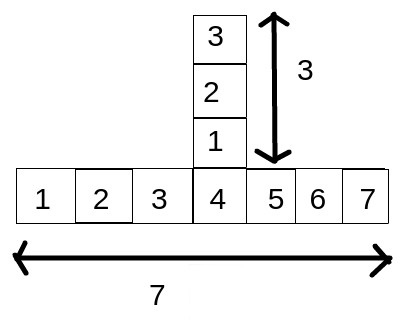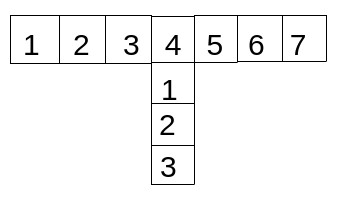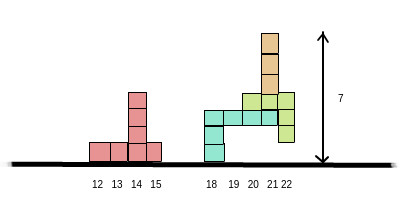Blocks
/

## medium

Problem
Editorial
Analytics

In this problem, we are going to build a structure using blocks of certain shape and size.

the block shape and size is described by the following rules -

l - horizontal length of the block
h - height of vertical block attached to the horizontal block
p - position on the horizontal block where the vertical block is attached
c - whether the vertical block is attached above the horizontal block or below it. 1 for above and 0 for below.

The width of the blocks is 1 unit.

See the figure for better explanation.

For l = 7 , h = 3 , p = 4 , c = 1For l = 7 , h = 3 , p = 4 , c = 0These blocks can be dropped vertically from above at horizontal positions - x (the position of the left of the block).

You need to calculate the final height of the structure. The final height is the maximum height over all horizontal positions.

Check Input/Output format and example for better understanding.

Constraints:-
1 ≤ n$5*10^{5}$
1 ≤ x$10^{5}$
1 ≤ l,p$10^{4}$
0 ≤ h$10^{4}$
0 ≤ c ≤ 1

Input Format:-
First line contain a single integer N denoting the number of blocks.
Next N lines follows -$i^{th}$ line consisting of specifications of the $i^{th}$ blocks and horizontal position of drop - l h p c x

Output Format:- Single Integer denoting the maximum height over all horizontal positions.

SAMPLE INPUT
4
4 3 3 1 12
4 2 1 0 18
3 2 3 0 20
1 2 1 1 21
SAMPLE OUTPUT
7
ExplanationTime Limit: 2.0 sec(s) for each input file.
Memory Limit: 256 MB
Source Limit: 1024 KB

## This Problem was Asked in

Initializing Code Editor...